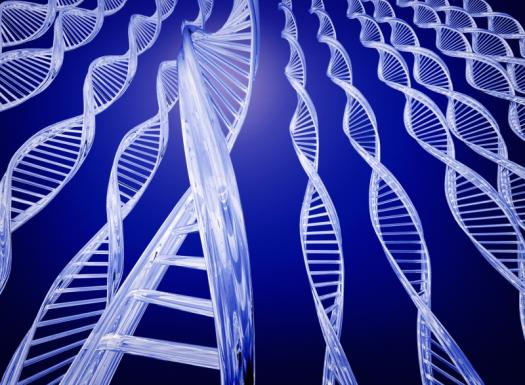# Introduction To Genes

7 Questions | Total Attempts: 87SettingsThis quiz will quiz you on what you know on Genes. Good luck : )

Related Topics
• 1.
Where did you get your genes?
• A.

The mall

• B.

• C.

• D.

Both B and C are correct

• 2.
How many chromosomes do we have?
• A.

12= total of 24

• B.

23= total of 46

• C.

20=total of 40

• D.

11 total of 22

• 3.
A _________trait is that which is weak and often does not show up.
• 4.
What is a dominant trait?
• A.

A gene in one strand of DNA that is stronger than the corresponding gene in another strand of DNA.

• B.

A trait that is weak and does not show up.

• C.

Both A and B

• 5.
Traits help determine
• A.

• B.

• C.

Hair color

• D.

Ear lobe shape

• E.

All of the above

• 6.
What are the four nucleotides called?
• A.

* adenine(A), * cytosine (C), * guanine (G), and * thymine (T).

• B.

The four nucleotides are called: * adenine(A), * cytosine (C), * guanine (G), and * thoride (T)

• C.

The four nucleotides are called: * adenine(A), * chloride (C), * guanine (G), and * thymine (T).

• D.

The four nucleotides are called: * ammonia (A) * cytosine (C), * guanine (G), and * thymine (T).

• E.

None of the above

• 7.
Where are chromosomes found within a living cell?
• A.

Outside the cell walls

• B.

Nucleus

• C.

Cell memebrane

• D.

In the ribosomes